# Law of Algebra of sets

Law of Algebra of sets:

a) Impotent law- AA=A and A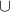A = A
b) Identity Law – A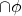= A and Au=A
c) Commutative law- AB = BA and AB = BA
d) Associative law- (AB)C = A(BC)
e) Distributive law- A(BC) = (AB)∩(AC)
– A(BC)= (AB)(AC)

Some Use Full Theorem

If A and B are any two sets then

a) A-B = A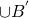b) B-A=B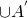c) A-B=A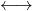AB =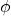d) (A-B)B = AB
e) (A-B)B =f) AB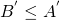g) (A-B)(B-A) = (AB) – (AB)

Some Important Result on Number of Elements In SETS
If A,B and C are finite sets and u be the universal set then.

1. n(AB) = n(A) + n(B) – n(AB)
2. n(AB) = n(A) + n(B) + n(B)A,B are adjoint non vaid sets.
3. n(A-B) = n(A) – n(AB)
i.e n(A-B) + n(AB) = n(A)
4. n(AB) = number of elements which belong to exactly one of A or B
= n(A-B)(B-A)
= n(A-B) +n(B-A) ……………. [(A-B) and (B-A) are disjoint]
= n(A)- n(AB) + n(B) – n(AB)
= n(A) + n(B) – 2n(AB)
5. n(ABC) =
n(A) + n(B) + n(c) – n(A∩B) –n(BC)-n(AC) + n(ABC)
6. number of elements in exactly two of the sets A,B,C
= n(AB) + n(BC) + n(CA)- 3n(ABC)
7. number of elements in exactly on cot the sets A,B,C
= n(A) + n(B) + n(c) – 2n(AB) –2n(BC)-2n(AC) + 3n(ABC)
8. n(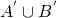) = n((A∩B)’) = n(u) – n(AB)

Certesian Product Of Sets

Ordered Pair– an ordered pair consists of two objects or elements in a given fixed order
If A&B are any two sets then by an order pair of elements are (a,b) Where aA and bB
The position of a paint in two dimensional plane intercession coordinate is represented by an order pair (-1,5) Where xR and Yr

CARTESIAN PRODUCT OF SETS: if A and B are two non empty sets the set of all ordered pairs (a,b) such as a aA and bB is called Cartesian product of the set A and B and is denoted by AB

A×B = {a,b}: aA and bB}

Example if A= (x,y) and B= (2,3,4) find A×B, B×A, A×A
A×B = {(x,z), (x,3), (x,4), (y,z), (y,3), (y,4)}
B×A= {(z,x), (z,y), (3,x), (3,y), (4,x), (4,y)}
A×A= {(x,x), (x,y), (y,x), (y,y)}
As above (A×B)(B×A) =Graphical Representation Of Cartesian Product Of Sets
If A = {2,3,4}
B = {3,4}
A×B = {(2,3) (2,4) (3,3) (3,4), (4,3), (4,4)}
To represent A×B graphical, draw to line perpendicular to each X & Y axis and then draw these pairs.
As above n(A×B) = n(A), n(B) = 2×3 = 6 Pair

A×B =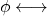A =, B =A×A×A= {(a,b,c) : a,b,c A}
(a,b,c) is called an ordered triplet

(i) A× (BUC) = (A×B)U (A×C)
(ii) A×(B∩C) = (A×B)∩ (A×C)
(iii) A×(B-C) = (A×B)- (A×C)
(iv) A×B = B×AA=B
(v) A≤B then A×A ≤ (A×B) (B×A)
(vi) A≤ B then A×C ≤ B×C
(vii) A≤B and C≤D than A×C ≤ B × D
(viii) For any set Four A,B,C,D
(A×B)∩ (C×D) = (A∩C) × (B∩D)
For any set A and B
(A×B)∩ (B×A) = (A∩B) × (B∩A)
(ix) For any three set A,B,C
A × (B’UC’) = (A×B) ∩(A×C)
A× (B’∩C’) = (A×B)U (A×C)
(x) If any two non empty sets have n elements in common. Then A×B and B×A have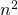elements in common.
(xi) If A is non empty set and A×B = A×C
Then B=C

Set relationship